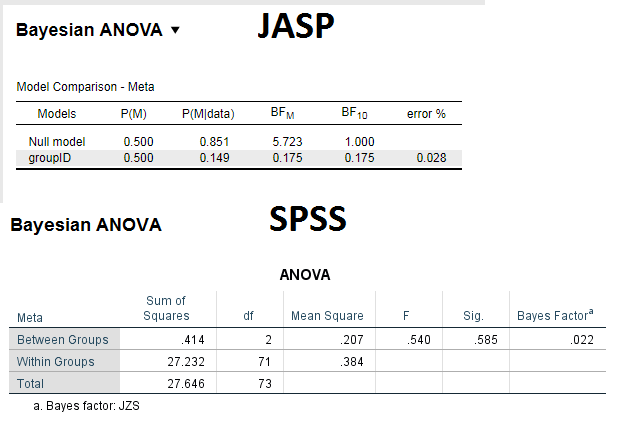#### Howdy, Stranger!

It looks like you're new here. If you want to get involved, click one of these buttons!

Supported by

# Interpreting results of one-way bayesian ANOVA in JASP

Dear forum,

I would like to ensure that I correctly interpret and report the results of one-way bayesian ANOVA (different samples, not repeated measures). I attach an output of my analysis (using JASP and SPSS). My questions are:
1. If I read the output correctly, in JASP I get Bayes factor (BF10) 0.175. Given that it is < 0.33, can I say that this supports H0?
2. When I report my JASP analysis I say that the priors were based on cauchy distribution. This is what I understood from this paper: https://link.springer.com/article/10.3758/s13423-017-1323-7 Would it be correct statement?
3. For comparison, I ran the same analysis in SPSS (Jeffreys–Zellner–Siow method). in SPSS the Bayes factor is 0.022. Is the discrepancy between two programs because of different priors?

Thank you a lot,1. You have BF10 = 0.175. This means that that BF01 = 1/0.175 = 5.71, meaning that the data are about 5.7 times more likely under H0 than under H1. This is support in favor of H0. I should not that BF10 = 0.99 would also have constituted evidence for H0 (but only a smidgen).
2. Yes, that is correct.
3. Weird. You can check with BayesFactor. I'll discuss this with Richard too. I doubt SPSS gives the correct result, because that BF is very small indeed.

Cheers,
E.J.

• SPSS is in error, almost surely. It would require a prior scale of almost two to get that low a Bayes factor.

Here's my check. This isn't exactly right, because it appears you have slightly different numbers in each group, but this is the only way I can just use the F statistic. It should be very close.

``````Fstat = .540
N = 25
J = 3

df1 = J - 1
df2 = (N - 1) * J

1 - pf(.540, df1, df2 )
###  0.5850897
### P value checks out against SPSS

bf = BayesFactor::oneWayAOV.Fstat(Fstat, N, J, simple = TRUE)
###       B10
### 0.1730758
### Agrees with JASP

### Check for a range of prior scales
rscale = exp(seq(log(.1), log(2), len = 50))
bfs = sapply(rscale, function(r)
BayesFactor::oneWayAOV.Fstat(Fstat, N, J, simple = TRUE, rscale = r))

plot(rscale, bfs, ty='l', log = "xy", ylab = "Bayes factor", xlab = "Prior scale", las = 1)

## Blue line is default
abline(h = bf, col = "blue")
abline(v = 1/2, col = "blue")

## Red line is SPSS - way out there.
abline(h = .022, col = "red")

``````

• Hello everyone,

First, many thanks to the JASP team for the amazing job! I have the following naive questionFor a study we did 4 one-way Bayesian ANOVAs (for 4 different tasks) for both Reaction times and Accuracies. For the Acc the BF10 and 01 are easy to interpret (meaning that there is clear support either for BF10 or BF01), but for the RTs both the BF01 and BF10 are negligible (less than 1). So my question is: when both BFs are less than 1 would it be correct statement to say that the data (in this case the RT data) is not informative ( probably more evidence is needed) and thus no reliable conclusion can be provided by from this data? Or some other way to handle such small BF10 and BF01?

• Hi Mila,

BF10 = 1/BF01, so if BF10 < 1 then BF01 > 1. In other words, it is impossible that both BF10 and BF01 are lower than 1, unless you are referring to different tests. Maybe you have a concrete example? In general, BFs near 1 are not diagnostic, at least not as far as the hypotheses are concerned that you are testing.

Cheers,
E.J.

• Hi E.J.,
Thanks for the response. Indeed, you are right I dis not express muself correctly. What I was wondering was wheter for cases like BF10 = 0.956 and BF01 = 1.046, for example, where both BFs are not diagnostic, as you said, would be sufficient to say that the data is not sensitive with respect to hypotheses tested and refrain from any conclusions?
Thanks again!
• Hi Mila,

Yes. The correct conclusion is that the data do not provide information: the hypotheses under consideration predicted the data about equally well. Of course, the data may be informative in other ways; for instance, perhaps the posterior distribution is relatively peaked, or perhaps most posterior mass is on positive values of effect size, etc.

Cheers,
E.J.

• Many thanks!